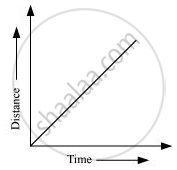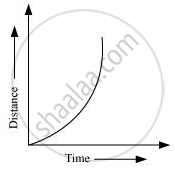Share

# What is the Nature of the Distance-time Graphs for Uniform and Non-uniform Motion of an Object? - CBSE Class 9 - Science

ConceptGraphical Representation of Motion - Distance-time Graphs

#### Question

What is the nature of the distance-time graphs for uniform and non-uniform motion of an object?

#### Solution

The distance-time graph for uniform motion of an object is a straight line (as shown in the following figure).The distance-time graph for non-uniform motion of an object is a curved line (as shown in the given figure).Is there an error in this question or solution?

#### Video TutorialsVIEW ALL 

Solution What is the Nature of the Distance-time Graphs for Uniform and Non-uniform Motion of an Object? Concept: Graphical Representation of Motion - Distance-time Graphs.
S